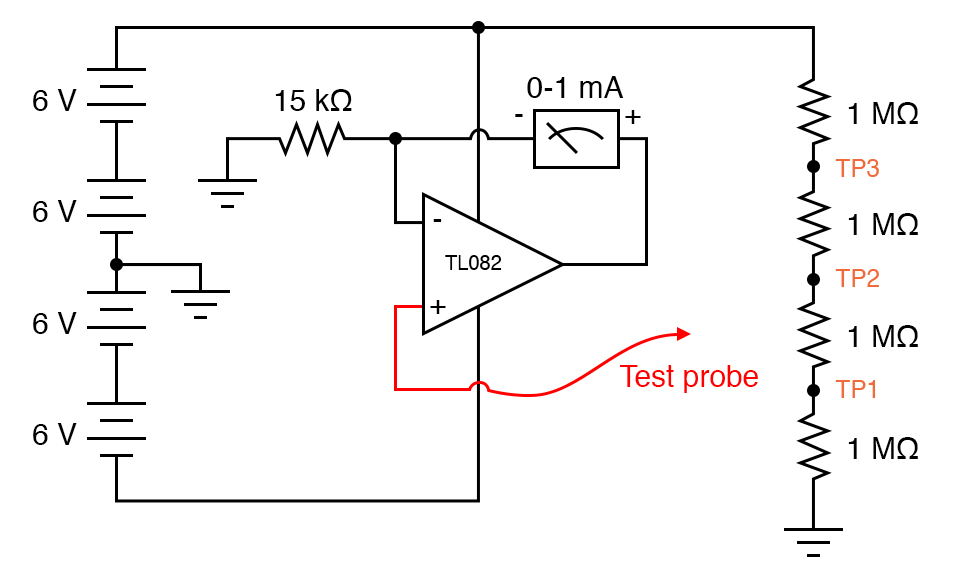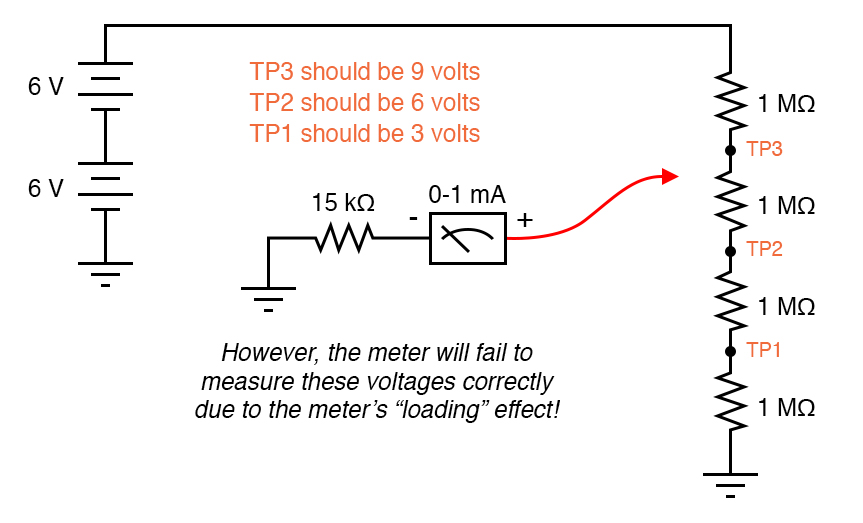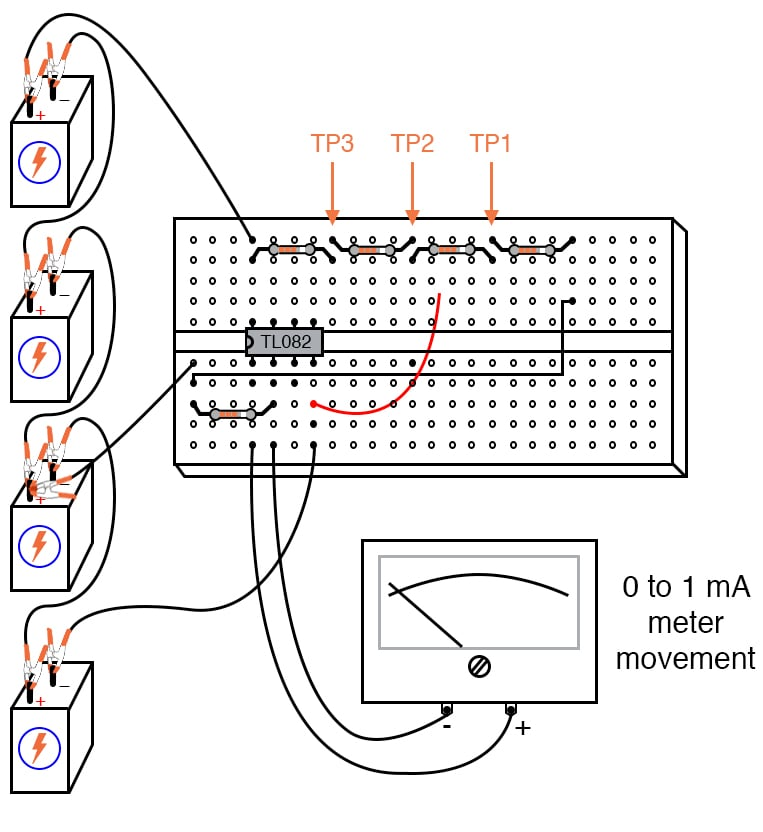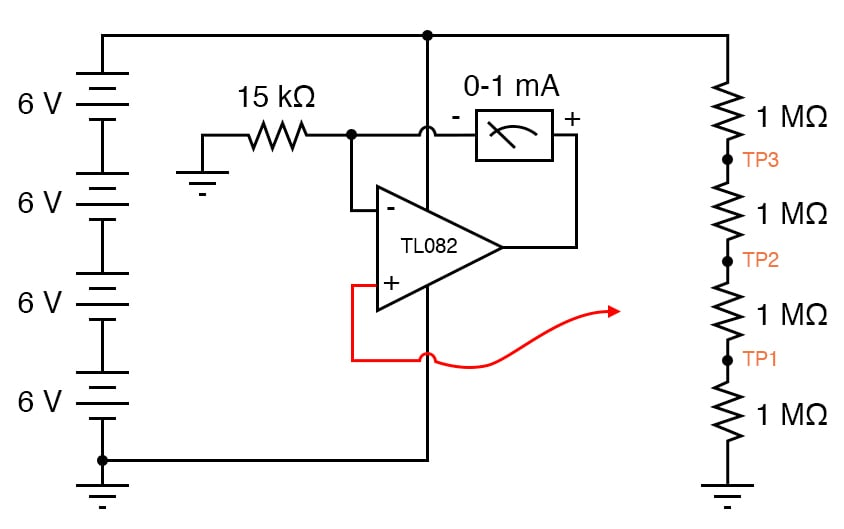Vol. DIY Electronics Projects
Chapter 6 Analog IC Projects

# Analog Lab - High-impedance Voltmeter

### Project Overview

An ideal voltmeter has infinite input impedance, drawing zero current from the circuit under test. This way, there will be no impact on the circuit as the voltage is being measured. The more current a voltmeter draws from the circuit under test, the more the measured voltage will sag under the loading effect of the meter. This is similar to a tire-pressure gauge releasing air out of the tire being measured: the more air released from the tire, the more the tire’s pressure will be impacted by the act of measurement.

This loading is more pronounced on high-resistance circuits, like the voltage divider made of 1 MΩ resistors, shown in Figure 1.### Parts and Materials

• An operational amplifier (op amp), model TL082 (datasheet) recommended
• An operational amplifier, model LM1458 (datasheet) recommended
• Four 6 V or 24 V power supply
• 15 VDC, 1mA analog panel meter
• 15 kΩ precision resistor
• Four 1 MΩ resistors

This second main portion of this experiment is based on junction field-effect transistors or JFET-input op amp such as the TL082 and is designed to demonstrate an issue known as latch-up. The other op amp (model 1458) is used in this experiment to demonstrate the absence of latch-up, a problem inherent to the TL082. If you do not have a JFET-input opamp, you can skip the latch-up portion of the experiment.

You don’t need the 1 MΩ resistors, exactly. Any very high-resistance resistors will suffice.

### Learning Objectives

• To show how to make a high-impedance voltmeter using an op amp
• To illustrate what op amp latch-up is and how to avoid it

### Instructions

Step 1: Build a simple 0-15 V range voltmeter by connecting the 1 mA meter movement in series with the 15 kΩ precision resistor, as illustrated in Figure 2.Step 2: Try to use this voltmeter to measure the voltage at TP1, TP2, or TP3 (with respect to ground). You will encounter severe measurement errors induced by meter impact. Does the meter read falsely high or falsely low? Why do you think this is?

Step 3: If we were to increase the meter’s input impedance, we would diminish its current draw or load on the circuit under test and consequently improve its measurement accuracy. An op amp with high-impedance inputs (using a JFET transistor input stage rather than a bipolar junction transistor (BJT) input stage) works well for this application.

Build the high-impedance voltmeter defined in the schematic diagram of Figure 1 and illustrated in Figure 3.##### Figure 3. Breadboard implementation of the high-impedance voltmeter circuit.

Note that the meter movement is part of the op amp’s feedback loop from the output to the inverting input. This circuit drives the meter movement with a current proportional to the voltage impressed at the noninverting (+) input. This current is supplied directly from the batteries through the op amp’s power supply pins, not from the circuit under test through the test probe. The meter’s range is set by the resistor connecting the inverting (-) input to the ground.

Step 4: Re-take voltage measurements at TP1, TP2, and TP3. You should enjoy far better success this time, with the meter movement accurately measuring these voltages (approximately 3, 6, and 9 V, respectively).

You may witness the extreme sensitivity of this voltmeter by touching the test probe with one hand and the most positive battery terminal with the other. Notice how you can drive the needle upward on the scale simply by measuring battery voltage through your body resistance, an impossible feat with the original, unamplified voltmeter circuit. If you touch the test probe to the ground, the meter should read exactly 0 V.

#### Latch-up in Integrated Circuits

If you do not have the TL082 or similar op amp with JFET op amps, you can skip the rest of this experiment.

Step 5: After you’ve proven this circuit to work, modify it by changing the power supply from dual rail (±12 V) to single-ended (+24 V). This entails removing the center-tap ground connection between the 2nd and 3rd batteries and grounding the far negative battery terminal instead, as shown in Figure 4.##### Figure 4. High-impedance voltmeter circuit with a single-ended power supply.

Step 6: Measure the voltages at TP1, TP2, and TP3. This alteration in the power supply increases the voltages at TP1, TP2, and TP3 to 6, 12, and 18 V, respectively. With a 15 kΩ range resistor and a 1 mA meter movement, measuring 18 V will gently peg the meter, but you should be able to measure the 6 and 12 V test points just fine.

Step 7: Try touching the meter’s test probe to the ground. This should drive the meter needle to exactly 0 V, as before, but it will not! What is happening here is an op amp phenomenon called latch-up, where the op amp output drives to a positive voltage when the input common-mode voltage exceeds the allowable limit.

In this case, as with many JFET-input op amps, neither input should be allowed to come close to either power supply rail voltage. With a single supply, the op amp’s negative power rail is at ground potential (0 V), so grounding the test probe brings the noninverting (+) input exactly to that rail voltage. This is bad for a JFET op amp, and drives the output strongly positive, even though it doesn’t seem like it should, based on how op amps are supposed to function.

When the op amp operated from a dual supply (+12/-12 V, rather than a single +24 V supply), the negative power supply rail was 12 V away from the ground (0 V), so grounding the test probe didn’t violate the op amp’s common-mode voltage limit.

However, with the single +24 V supply, we have a problem. Note that some op amps do not latch-up the way the model TL082 does.

Step 8: Finally, you may replace the TL082 with an LM1458 op amp, which is pin-for-pin compatible (no breadboard wiring changes needed). Model 1458 will not latch-up when the test probe is grounded, although you may still get incorrect meter readings with the measured voltage exactly equal to the negative power supply rail. As a general rule, you should always be sure the op amp’s power supply rail voltages exceed the expected input voltages.

### Related Content

Textbook:

Worksheet:

#### Lessons in Electric Circuits

Published under the terms and conditions of the Design Science License